Search IntMath
Close

450+ Math Lessons written by Math Professors and Teachers

5 Million+ Students Helped Each Year

1200+ Articles Written by Math Educators and Enthusiasts

Simplifying and Teaching Math for Over 23 Years

# 7. Vectors in 3-D Space

We saw earlier how to represent 2-dimensional vectors on the x-y plane.

Now we extend the idea to represent 3-dimensional vectors using the x-y-z axes. (See The 3-dimensional Co-ordinate System for background on this).

### Example

The vector OP has initial point at the origin O (0, 0, 0) and terminal point at P (2, 3, 5). We can draw the vector OP as follows: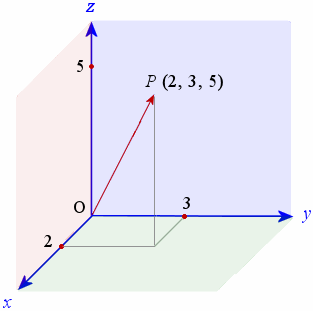## Magnitude of a 3-Dimensional Vector

We saw earlier that the distance between 2 points in 3-dimensional space is

"distance"\ AB =  sqrt ((x_2-x_1)^2+ (y_2-y_1)^2+ (z_2-z_1)^2)

For the vector OP above, the magnitude of the vector is given by:

| OP | = sqrt(2^2+ 3^2+ 5^2) = 6.16\ "units"

Earlier we saw how to add 2-dimensional vectors. We now extend the idea for 3-dimensional vectors.

We simply add the i components together, then the j components and finally, the k components.

### Example 1Two anchors are holding a ship in place and their forces acting on the ship are represented by vectors A and B as follows:

A = 2i + 5j − 4k and B = −2i − 3j − 5k

If we were to replace the 2 anchors with 1 single anchor, what vector represents that single vector?

The problem just requires us to add the vectors to get the single resultant vector.

 A + B = (2 + −2) i + (5 − 3)j + (−4 −5)k = 0 i + 2 j − 9 k = 2 j − 9 k

## Dot Product of 3-dimensional Vectors

To find the dot product (or scalar product) of 3-dimensional vectors, we just extend the ideas from the dot product in 2 dimensions that we met earlier.

### Example 2 - Dot Product Using Magnitude and Angle

Find the dot product of the vectors P and Q given that the angle between the two vectors is 35° and

| P | = 25 units and | Q | = 4 units

Using our formula from before for dot product:

P • Q = |P| |Q| cos θ

we have:

 P • Q = |P| |Q| cos θ = 25 × 4 × cos 35° = 81.92

### Example 3 - Dot Product if Vectors are Multiples of Unit Vectors

Find the dot product of the vectors A and B (these come from our anchor example above):

A = 2i + 5j − 4k and B = −2i − 3j − 5k

 A • B = (2i + 5j − 4k) • (−2i − 3j − 5k) = (2 × −2) + (5 × −3) + (−4 × −5) = −4 + −15 + 20 = 1

## Direction Cosines

Suppose we have a vector OA with initial point at the origin and terminal point at A.

Suppose also that we have a unit vector in the same direction as OA. (Go here for a reminder on unit vectors).

Let our unit vector be:

u = u1 i + u2 j + u3 k

On the graph, u is the unit vector (in black) pointing in the same direction as vector OA, and i, j, and k (the unit vectors in the x-, y- and z-directions respectively) are marked in green.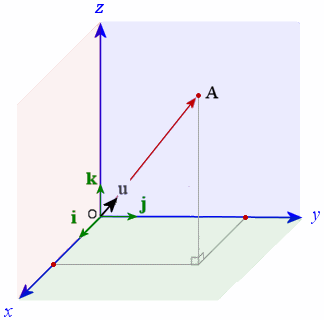We now zoom in on the vector u, and change orientation slightly, as follows: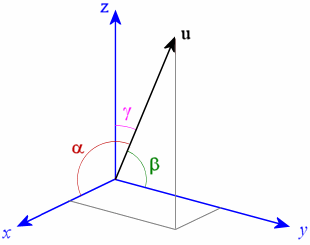Now, if in the diagram above,

α is the angle between u and the x-axis (in dark red),
β
is the angle between u and the y-axis (in green) and
γ
is the angle between u and the z-axis (in pink),

then we can use the scalar product and write:

 u1 = u • i = 1 × 1 × cos α = cos α
 u2 = u• j = 1 × 1 × cos β = cos β
 u3 = u • k = 1 × 1 × cos γ = cos γ

So we can write our unit vector u as:

u = cos α i + cos β j + cos γ k

These 3 cosines are called the direction cosines.

## Angle Between 3-Dimensional Vectors

Earlier, we saw how to find the angle between 2-dimensional vectors. We use the same formula for 3-dimensional vectors:

theta=arccos((P * Q)/(|P||Q|))

### Example 4

Find the angle between the vectors P = 4i + 0j + 7k and Q = -2i + j + 3k.

The vectors P and Q are as follows. Vector P is on the x-z plane (note that the y-value for vector P is 0) , while Q is 'behind' the y-z plane.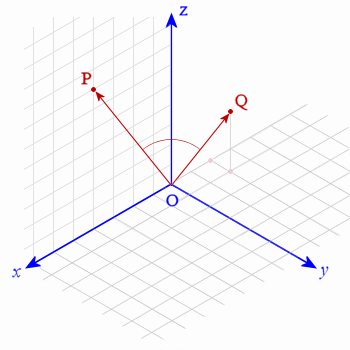Using the formula

theta=arccos((P*Q)/(|P||Q|))

we have:

 P • Q = (4 i + 0 j + 7 j) • (−2 i + j + 3 k ) = (4 × −2) + (0 × 1) + (7 × 3) = 13

And now for the denominator:

|P||Q|= sqrt (4^2+ (0)^2+ 7^2) xxsqrt((-2)^2+1^2+3^2)

= sqrt (65)sqrt(14)

=30.166\ "units"

So

θ = arccos(13 ÷ 30.166)

Therefore the angle between the vectors P and Q is

θ = 64.47°

### Exercise

Find the angle between the vectors P = 3i + 4j − 7k and Q = -2i + j + 3k.

Using the formula

theta=arccos((P*Q)/(|P||Q|))

we find the dot product first:

 P • Q = (3 i + 4 j − 7 j) • (−2 i + j + 3 k ) = (3 × −2) + (4 × 1) + (−7 × 3) = −23

And now for the denominator:

 |P| |Q| = √(32 + 42 + (−7) 2) × √((−2)2 + 12 + 32) = 32.187

So

θ = arccos(−23 ÷ 32.187)

Therefore the angle between the vectors P and Q is

θ = 135.6°

### Application

We have a cube ABCO PQRS which has a string along the cube's diagonal B to S and another along the other diagonal C to P

What is the angle between the 2 strings?

For convenience, we will assume that we have a unit cube (each side has length 1 unit) and we place it such that one corner of the cube is at the origin.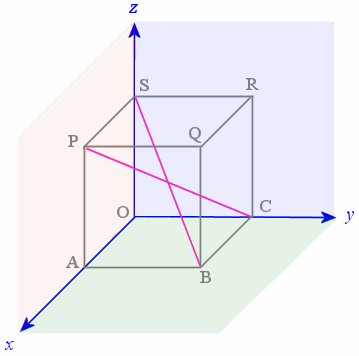The unit vectors i, j, and k act in the x-, y-, and z-directions respectively. So in our diagram, since we have a unit cube,

OA = i
OC = j
OS = k

From the diagram, we see that to move from B to S, we need to go −1 unit in the x direction, −1 unit in the y-direction and up 1 unit in the z-direction. Since we have a unit cube, we can write:

BS = −i − j + k

and similarly:

CP = i − j + k

The scalar product for the vectors BS and CP is:

BS • CP = |BS| |CP| cos θ

where θ is the angle between BS and CP.

So the angle θ is given by

θ = arccos[ (BS • CP) ÷ ( |BS| |CP| ) ]

Now,

BS • CP

= (−i − j + k) (i − j + k)

= 1 + 1 + 1

= 1

and

|BS| |CP|

= sqrt((-1)^2 + (-1)^2 + 1^2)  × sqrt(1^2 + (-1)^2 + 1^2)

= (sqrt3)(sqrt3)

= 3

So

θ = arccos (1/3)

θ = 70.5°

So the angle between the strings is 70.5°. (In this situation we assume "angle" refers to the acute angle between the strings.)

# Problem Solver

Need help solving a different Graphing problem? Try the Problem Solver.

Disclaimer: IntMath.com does not guarantee the accuracy of results. Problem Solver provided by Mathway.